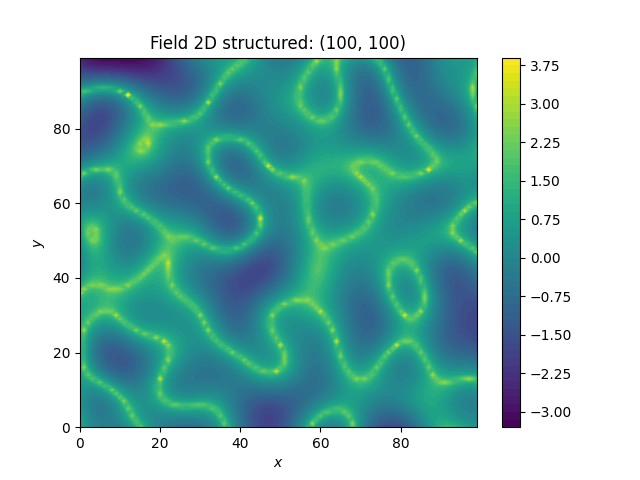# Zinn & Harvey transformation¶

Here, we transform a field with the so called “Zinn & Harvey” transformation presented in Zinn & Harvey (2003). With this transformation, one could overcome the restriction that in ordinary Gaussian random fields the mean values are the ones being the most connected.```import gstools as gs

# structured field with a size of 100x100 and a grid-size of 1x1
x = y = range(100)
model = gs.Gaussian(dim=2, var=1, len_scale=10)
srf = gs.SRF(model, seed=20170519)
srf.structured([x, y])
gs.transform.zinnharvey(srf, conn="high")
srf.plot()
```

Total running time of the script: ( 0 minutes 1.155 seconds)

Gallery generated by Sphinx-Gallery